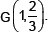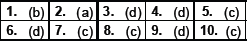# Coordinate Geometry-MCQ

Class X Math
MCQ for Coordinate Geometry
1.   Find the coordinates of the point equidistant from the points A(1, 2), B (3, –4) and C(5, –6).
(a) (2, 3)
(b) (–1, –2)
(c) (0, 3)
(d) (1, 3)
2.   Find the coordinates of the point equidistant from the points A(5, 1), B(–3, –7) and C(7, –1).
(a) (2, –4)
(b) (3, –6)
(c) (4, 7)
(d) (8, –6)
3.   Two of the vertices of a ��ABC are given by A(6, 4) and B(–2, 2) and its centroid is G(3, 4). Find the coordinates of the third vertex C of the ΔABC.
(a) (2, 3)
(b) (4, 6)
(c) (4, 3)
(d) (5, 6)
4.   Find the value of P for which the point (–1, 3), (2, p) and (5, –1) are collinear.
(a) 4
(b) 3
(c) 2
(d) 1
5.   Find the distance of the point (–6, 8) from the origin.
(a) 8
(b) 11
(c) 10
(d) 9
6.   Find the value of p for which the points (–5, 1), (1, p) and (4, –2) are collinear.
(a) –3
(b) –2
(c) 0
(d) –1
7.   Find the value of k if the points A(2, 3), B(4, k) and C(6, –3) are collinear.
(a) 2
(b) 3
(c) 0
(d) 1
8.   In what ratio of line x – y – 2 = 0 divides the line segment joining (3, –1) and (8, 9)?
(a) 1 : 2
(b) 2 : 1
(c) 2 : 3
(d) 1 : 3
9.   The vertices of a ΔABC and given by A(2, 3) and B(–2, 1) and its centroid is GFind the coordinates of the third vertex C of the ΔABC.
(a) (0, 2)
(b) (1, –2)
(c) (2, –3)
(d) (–2, 3)
10.   Find the ratio in which the line joining the points (6, 4) and (1, –7) is divided by x-axis.
(a) 1 : 3
(b) 2 : 7
(c) 4 : 7
(d) 6 : 7